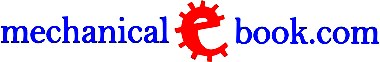Custom Search
 A B C D E F G H I J K L M N O P Q R S T U V W X Y Z
 Transformer Transformers are used for stepping up alternating current to high voltages for long-distance power transmission in order to minimize the relative voltage losses and also for stepping down the voltage at the point of consumption. A transformer is a device that transfers electrical energy from one circuit to another through inductively coupled electrical conductors. A changing current in the first circuit (the primary) creates a changing magnetic field; in turn, this magnetic field induces a changing voltage in the second circuit (the secondary). By adding a load to the secondary circuit, one can make current flow in the transformer, thus transferring energy from one circuit to the other. Electrical transformers are used to transform voltage from one level to another, usually from a higher voltage to a lower voltage. They do this by applying the principle of magnetic induction between coils to convert voltage and/or current levels. In this way, electrical transformers are a passive device which transforms alternating current (otherwise known as AC) electric energy from one circuit into another through electromagnetic induction. An electrical transformer normally consists of a ferromagnetic core and two or more coils called windings. A changing current in the primary winding creates an alternating magnetic field in the core. In turn, the core multiplies this field and couples the most of the flux through the secondary tranformer windings. This in turn induces alternating voltage (or emf) in each of the secondary coils. The common operation of a transformer is closely associated with Faraday’s law of induction, which defines that when the magnetic flux enclosed within a circuit varies, an electric current which is proportional to the rate of variation is induced in the circuit. If the magnetic flux variation is produced by means of an electromagnet coil energized by alternating (primary winding of the transformer), an induced electric current can be obtained from a second coil (secondary winding of the transformer) through which the varying magnetic flux from the first coil is made to pass. The two coils, i.e., the primary and the secondary winding, are mounted on the same iron core, so as to obtain maximum concentration of the flux. Transformation is characterized by its very high efficiency, practically the only energy losses related with low-frequency alternating current being heat losses, namely, heat developed in the resistance of the copper windings (copper losses) and heat due to eddy currents set up in the iron core. To minimize the eddy a current, the core is laminated, i.e., it is made up of a number of thin iron plates which are insulated from one another. The iron losses additionally comprise so called hysteresis losses. Ideally: U1.I1 = U2.I2 (Input voltage x input current = output voltage x output current). To increase the voltage, the secondary winding of the transformer has a larger number of turns of wire than the primary winding. To decrease the voltage before the current is supplied to the consumer; a transformer is used whose primary winding contains a large number of turns than the secondary winding. For local distribution in a town the high voltage of the power grid is transformed down to 6000 volts and this is in turn is reduced to 220 1 volts for distribution to individual consumers within the limited area served by the final step-down transformer. A low-voltage device such as an electric bell often obtains its electricity from the mains through a small transformer of its own (called a bell transformer) which gives current at 4-8 volts. Such a transformer is generally termed as an auto-transformer, which has only one winding, part of which forms the secondary, while the whole forms the primary, or vice versa. Its principle is illustrated in Figs.3a and 3b. It is cheaper in construction than a transformer with two windings, and the copper losses are lower. The ignition coil of an internal combustion engine operates on the same principle, except that it is a step-up transformer which has to deliver a high voltage for the sparking plugs. In this case the low voltage is applied to part of the winding and high voltage is taken from the whole winding. A commonly employed type of transformer is wound in concentric form, the primary winding being within the secondary. The iron core forms a double closed magnetic circuit. For practical purposes this is a more efficient design than the so-called toroidal transformer in Fig.1, which merely serves to illustrate the principle. o DISCLAIMER         o CONTACT US